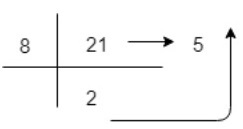# Octal Number Systemn

Octal Number System is one the type of Number Representation techniques, in which there value of base is 8. That means there are only 8 symbols or possible digit values, there are 0, 1, 2, 3, 4, 5, 6, 7. It requires only 3 bits to represent value of any digit. Octal numbers are indicated by the addition of either an 0o prefix or an 8 suffix.

Position of every digit has a weight which is a power of 8. Each position in the Octal system is 8 times more significant than the previous position, that means numeric value of an octal number is determined by multiplying each digit of the number by the value of the position in which the digit appears and then adding the products. So, it is also a positional (or weighted) number system.

## Representation of Octal Number

Each Octal number can be represented using only 3 bits, with each group of bits having a distich values between 000 (for 0) and 111 (for 7 = 4+2+1). The equivalent binary number of Octal number are as given below −

Octal Digit ValueBinary Equivalent
0000
1001
2010
3011
4100
5101
6110
7111

Octal number system is similar to Hexadecimal number system. Octal number system provides convenient way of converting large binary numbers into more compact and smaller groups, however this octal number system is less popular.

Most Significant Bit (MSB)Octal PointLeast Significant Bit (LSB)
8281808-18-28-3
64811/81/641/512

Since base value of Octal number system is 8, so there maximum value of digit is 7 and it can not be more than 7. In this number system, the successive positions to the left of the octal point having weights of 80, 81, 82, 83 and so on. Similarly, the successive positions to the right of the octal point having weights of 8-1, 8-2, 8-3and so on. This is called base power of 8. The decimal value of any octal number can be determined using sum of product of each digit with its positional value.

Example-1 − The number 111 is interpreted as

111 = 1x82+5x81+7x80 = 157

Here, right most bit 7 is the least significant bit (LSB) and left most bit 1 is the most significant bit (MSB).

Example-2 − The number 65.125 is interpreted as

65.125 =1x82+0x81+1x80+1x8-1=101.10

Here, right most bit 0 is the least significant bit (LSB) and left most bit 1 is the most significant bit (MSB).

Example-3 − A decimal number 21 to represent in Octal representation(21)10=2x81+5x80=(25)8
So, decimal value 21 is equivalent to 25 in Octal Number System.

## Applications of Octal Number System

The octal numbers are not as common as they used to be. However, Octal is used when the number of bits in one word is a multiple of 3. It is also used as a shorthand for representing file permissions on UNIX systems and representation of UTF8 numbers, etc.